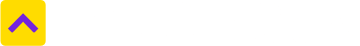# Convert Square Feet to Square Meter

One Square Feet is equal to 0.09290303997 Square Meter
Select State
Select from unit
Square Feet
Select to unit
0.09290303997
Square Meter

Defined as the area of a square with sides of 1 foot, a square foot is a non-metric unit commonly used across the world to measure property, mainly apartments and flats. Square foot has several abbreviations, including sq.ft., sf and ft2, while the term square feet is used to denote plural.

Across geographies, square foot, an imperial unit used to measure area, is used to map the area at the time of property purchases. Countries where the usage of square foot is most common include India, the US, the UK, Bangladesh, Canada, Ghana, Hong Kong, Liberia, Malaysia, Myanmar, Nepal, Pakistan and Singapore.

Use of this measurement unit is most common in architecture and real estate. This is the most commonly used unit to denote space in real estate in India, where the area of apartment units is typically provided in square foot terms and the cost is calculated based on this unit of measurement. While purchasing an apartment, buyers must make sure the square footage mentioned by the developer/seller verbally is actually correct. These days, sizes of homes are calculated using laser devices, it is better to err on the side of caution and doing you own due diligence to avoid property frauds.

Considering the square foot is a non-metric unit, the linear-unit conversion factor must be squared when converting between square foot and metric units of area such as meters squared or square centimeters.

 1 sqft 144 square inches 1 sqft 0.11 square yards 1 sqft 0.0929 square meters

### What are the most common land measurement units in India?

Hectares, acres, square meters and square yards are some of the common land measurement units used across India. While Bigha and Marla are commonly used in the North, terms such as Cent, Guntha and Ground belong to the South. Depending upon the names of the units, their sizes also vary from State to State.

### How much is Square Meter in Square Feet terms?

One Square Meter is equal to 10.76391042 Square Feet. Click here to convert

### How much is Square Feet in Square Meter terms?

One Square Feet is equal to 0.09290303997 Square Meter.
Trusted by
Partners© 2012-21 Locon Solutions Pvt. Ltd.